Physic Tutorials

# Notes on application Physics for Cambridge A level students

Notes on application Physics for Cambridge A level students

ELECTRONIC SENSORSENSING UNIT : This produce input voltage to processor depending on change in physical properties e.g temperatue, light or pressure.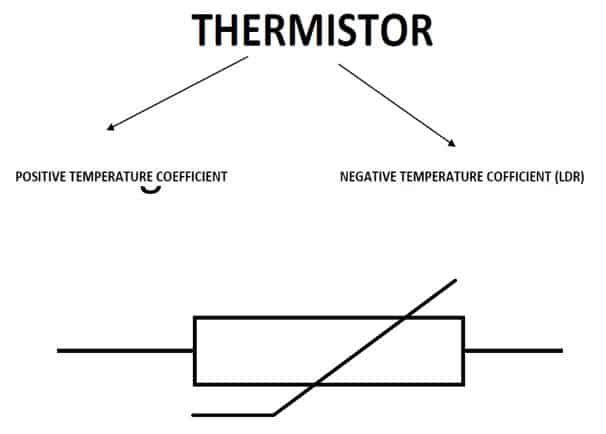Piezo electric Transducer

1. It converts energy from one form to another e.g microphone
2. Quartz, a crystal has its positive and negative ion joined at the centre
3. When pressure is applied the shape of the crystal changes
4. ons move apart to set the P.D which is amplify as output
5. Voltage is positive when pressure is above the ambient pressure
6. Voltage is negative when pressure is below the ambient pressureOUTPUT DEVICES

• RELAY
• LIGHT EMMITTING DIODE
• CALIBRATED DEVICES

RELAY

• A relay is an electromagnetic switch that uses a small current to switch on or off a larger current.
• It is also used to switch on large voltages by means of small voltages
• Isolate circuit from high voltage
• Remote switchingLIGHT EMMITTING DIODE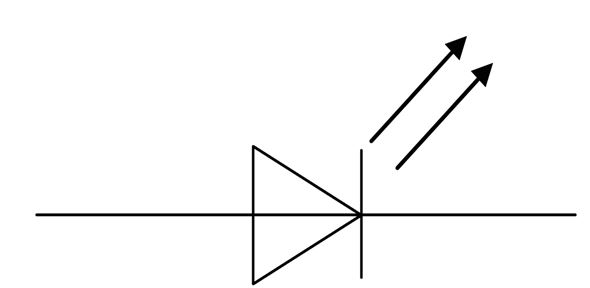PROCESSING UNITS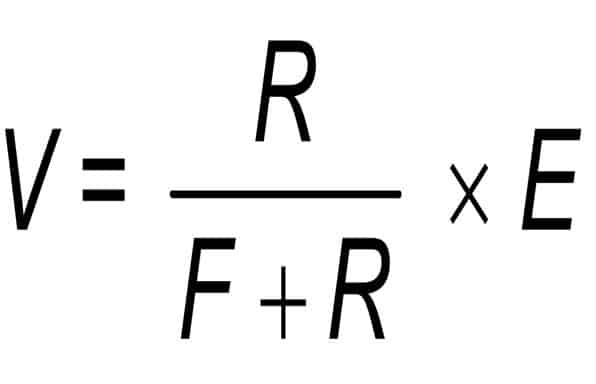Vout = A0 (V^+ – V^–)

where A0 is the open-loop gain of the op-amp

The ideal operational amplifier (op-amp) has the following properties:

• infinite input impedance
• infinite open-loop gain
• zero output impedance
• infinite bandwidth
• infinite slew rate

MAJOR USES OF AN OPERATIONAL AMPLIFIER

1. Used as a comparator
2. Used as an inverting amplifier
3. Used as a non-inverting amplifier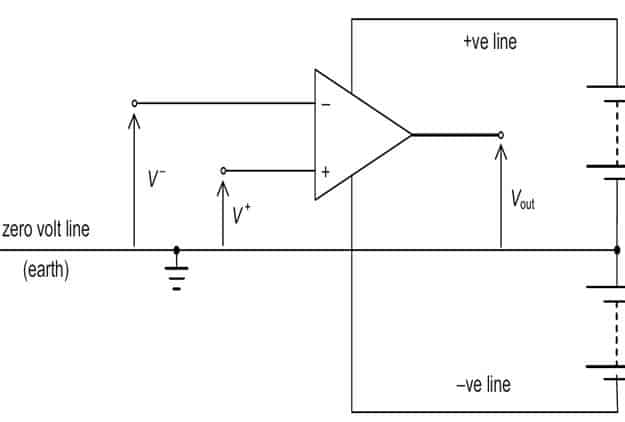NEGATIVE FEEDBACK
The process of taking some, or all, of the output of the amplifier and adding it to the input is known as feedback.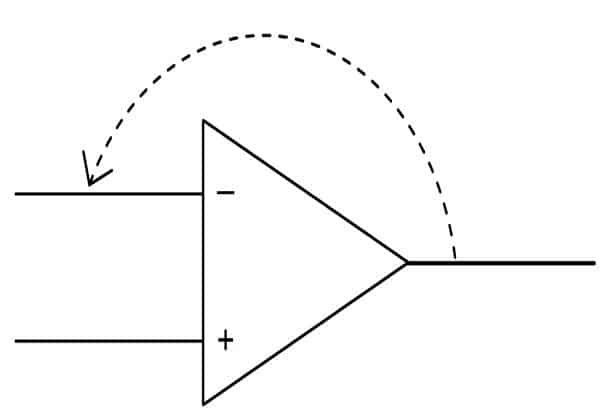• increased bandwidth,
• less distortion,
• greater operating stability

The circuit for an inverting amplifier is shown below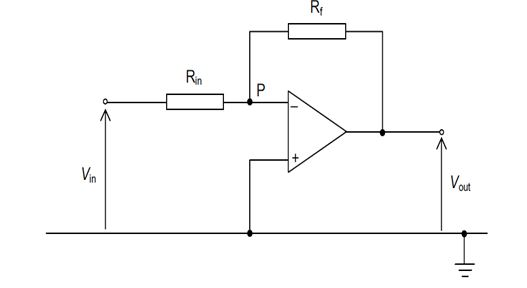An input signal Vin is applied to the input resistor Rin. Negative feedback is applied by means of the resistor Rf. The resistors Rin and Rf act as a potential divider between the input and the output of the operational amplifier.

Assumptions
There are two basic assumptions

In order that the amplifier is not saturated, the two input voltages must be almost the same. The non-inverting input (+) is connected directly to the zero-volt line (the earth) and so it is at exactly 0 V. Thus,

the inverting input (–) must be virtually at zero volts (or earth) and for this reason, the point P is known as a virtual earth.

The input impedance of the op-amp itself is very large, therefore, there is no current in either the non-inverting or the inverting inputs. It means the current in Rf is approximately equal to the current in RIN.

Recommended: Solved questions on capacitance

### Bolarinwa Olajire

A lecturer, Educationist, PhD student at FUNAAB, and a Blogger.